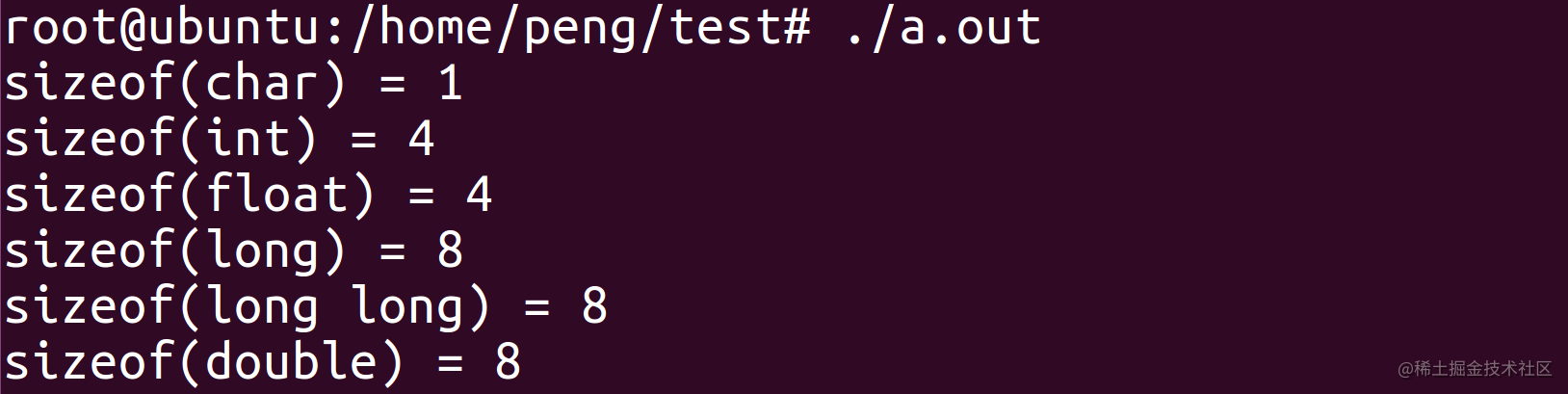# Linux字节对齐的那些事

## 一、概念

bit1个二进制位称为1个bit

word电脑用来一次性处理事务的一个固定长度

## 三、如何限制定字节对齐位数？

### 2. #pragma pack(n)

· 使用伪指令#pragma pack (n)，C编译器将按照n个字节对齐。 · 使用伪指令#pragma pack ()，取消自定义字节对齐方式。

#pragma pack(n) 用来设定变量以n字节对齐方式。 n字节对齐就是说变量存放的起始地址的偏移量有两种情况：

1. 如果n大于等于该变量所占用的字节数，那么偏移量必须满足默认的对齐方式
2. 如果n小于该变量的类型所占用的字节数，那么偏移量为n的倍数，不用满足默认的对齐方式。

### 3. 汇编.align

.align:用来指定数据的对齐方式,格式如下：

``````.align [absexpr1, absexpr2]

## 四、为什么要对齐？

``````unsigned int i = 0x12345678;
unsigned char *p=NULL;
unsigned short *p1=NULL;

p=&i;
*p=0x00;
p1=(unsigned short *)(p+1);
*p1=0x0000;

## 五、举例

### 例1：os基本数据类型占用的字节数

``````#include <stdio.h>

int main()
{
printf("sizeof(char) = %ld\n", sizeof(char));
printf("sizeof(int) = %ld\n", sizeof(int));
printf("sizeof(float) = %ld\n", sizeof(float));
printf("sizeof(long) = %ld\n", sizeof(long));
printf("sizeof(long long) = %ld\n", sizeof(long long));
printf("sizeof(double) = %ld\n", sizeof(double));
return 0;
}### 例2：结构体占用的内存大小--默认规则

``````struct yikou_s
{
double d;
char c;
int i;
} yikou_t;

``````sizeof(yikou_t) = 16

``````char: 偏移量必须为sizeof(char) 即1的倍数
int: 偏移量必须为sizeof(int) 即4的倍数
float: 偏移量必须为sizeof(float) 即4的倍数
double: 偏移量必须为sizeof(double) 即8的倍数

### 例3：调整结构体大小

``````struct yikou_s
{
char c;
double d;
int i;
} yikou_t;

``````sizeof(yikou_t) = 24

### 例4： #pragma pack(4)

``````#pragma pack(4)

struct yikou_s
{
char c;
double d;
int i;
} yikou_t;

``````sizeof(yikou_t) = 16

### 例5：#pragma pack(8)

``````#pragma pack(8)

struct yikou_s
{
char c;
double d;
int i;
} yikou_t;

``````sizeof(yikou_t) = 24

## 六、汇总实力

``````#include <stdio.h>
main()
{
struct A {
int a;
char b;
short c;
};

struct B {
char b;
int a;
short c;
};
struct AA {
// int a;
char b;
short c;
};

struct BB {
char b;
// int a;
short c;
};
#pragma pack (2) /*指定按2字节对齐*/
struct C {
char b;
int a;
short c;
};
#pragma pack () /*取消指定对齐，恢复缺省对齐*/

#pragma pack (1) /*指定按1字节对齐*/
struct D {
char b;
int a;
short c;
};
#pragma pack ()/*取消指定对齐，恢复缺省对齐*/

int s1=sizeof(struct A);
int s2=sizeof(struct AA);
int s3=sizeof(struct B);
int s4=sizeof(struct BB);
int s5=sizeof(struct C);
int s6=sizeof(struct D);
printf("%d\n",s1);
printf("%d\n",s2);
printf("%d\n",s3);
printf("%d\n",s4);
printf("%d\n",s5);
printf("%d\n",s6);
}